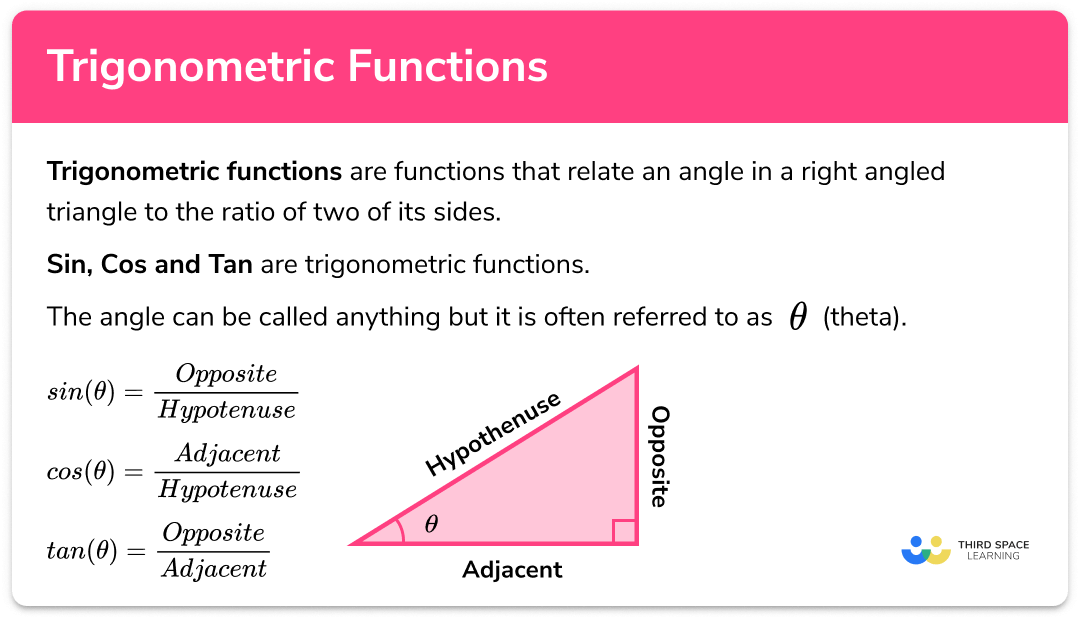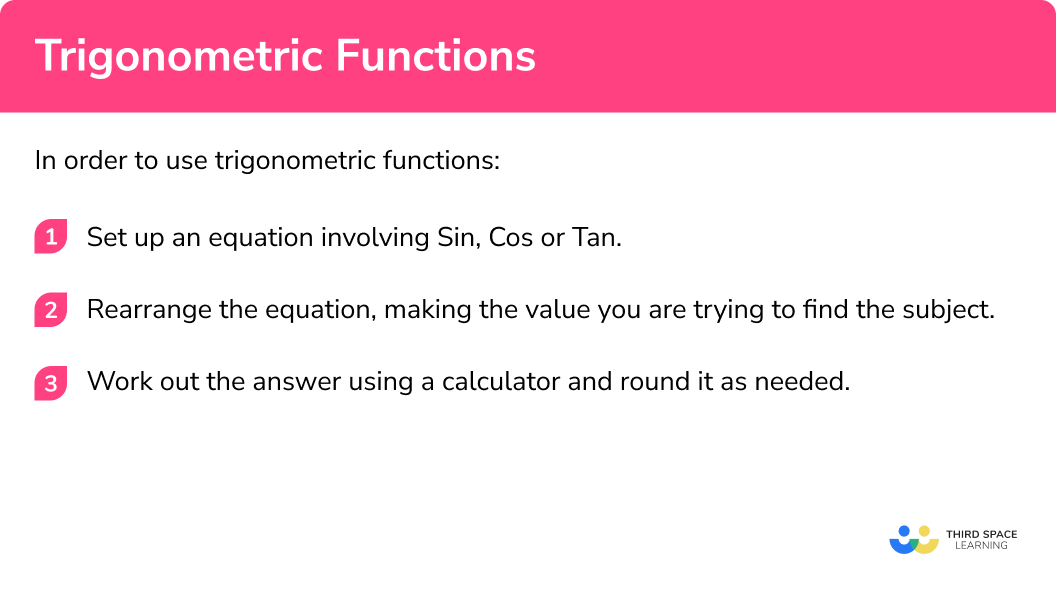GCSE Maths Geometry and Measure Trigonometry

Trigonometric Functions

# Trigonometric functions

Here we will learn what the trigonometric functions and inverse trigonometric functions are and how we can use them to calculate missing side lengths and angles in right-angled triangles.

Look out for the trigonometric functions practice problems, worksheets and exam questions at the end.

## What are trigonometric functions?

Trigonometric functions are functions that relate an angle in a right angled triangle to the ratio of two of its sides.

Sin, cos and tan are trigonometric functions.

The angle can be called anything but it is often referred to as ϴ (theta).

• \sin (\theta)

When we input a given angle into  \sin (\theta) it gives us the ratio between the opposite length and the hypotenuse.

$\sin ( \theta ) = \frac{Opposite}{Hypotenuse}$

It tells us how many times bigger the opposite is than the hypotenuse.

• \cos(\theta)

When we input a given angle into \cos(\theta) it gives us the ratio between the adjacent length and the hypotenuse.

$\cos ( \theta ) = \frac{Adjacent}{Hypotenuse}$

It tells us how many times bigger the adjacent is than the hypotenuse.

• \tan(\theta)

When we input a given angle into \tan(\theta) it gives us the ratio between the adjacent length and the opposite length.

$\tan ( \theta ) = \frac{Opposite}{Adjacent}$

It tells us how many times bigger the opposite is than the adjacent.

### What are trigonometric functions?### Sine, Cosine and Tangent

Trigonometric functions tell us how many times bigger one side is than another in triangles containing a right angle. Therefore, if we are given the length of one side and the value of one angle, we can set up equations using trigonometric functions to help us find the lengths of the other sides.

E.g.

• \sin(30)=\frac{x}{10}

We need to work out the value of x, to do this, we begin by writing an equation involving x.

$\text{We know }\sin(\theta)=\frac{Opposite}{Hypotenuse} \text{ therefore } \sin(30)=\frac{x}{10}$

Now we can rearrange this equation to make x the subject:

\begin{aligned} \sin(30)&=\frac{x}{10}\\ 10 \times \sin(30)&=x \end{aligned}

Finally we can type 10 × sin(30) into a calculator to find the value for x.

\begin{aligned} x&=10\times\sin(30)\\ x&=5cm \end{aligned}

• \cos(30)=\frac{x}{10}

$\text{We know }\cos(\theta)=\frac{Adjacent}{Hypotenuse} \text{ therefore } \cos(30)=\frac{x}{10}$

Now we can rearrange this equation to make x the subject:

\begin{aligned} \cos(30)&=\frac{x}{10}\\ 10 \times \cos(30)&=x \end{aligned}

Finally we can type 10 × cos(30) into a calculator to find the value for x.

\begin{aligned} x&=10\times\cos(30)\\ x&=8.660254038\\ x&=8.66cm (2dp) \end{aligned}

• \tan(30)=\frac{5}{x}

$\text{We know }\tan(\theta)=\frac{Opposite}{Adjacent} \text{ therefore } \tan(30)=\frac{5}{x}$

Now we can rearrange this equation to make x the subject:

\begin{aligned} \tan(30)&=\frac{5}{x}\\ x \times \tan(30)&=5\\ x&=\frac{5}{\tan(30)} \end{aligned}

Finally we can type \frac{5}{tan(30)} into a calculator to find the value for x .

\begin{aligned} x&=\frac{5}{\tan(30)}\\ x&=8.660254038\\ x&=8.66\mathrm{cm} ~(2\mathrm{dp}) \end{aligned}

## How to use trigonometric functions

In order to use trigonometric functions:

1. Set up an equation until involving sin, cos, or tan.
2. Rearrange the equation, making the value you are trying to find the subject.
3. Work out the answer using a calculator and round it as needed.

### How to use trigonometric functions## Trigonometric functions examples

### Example 1: calculating with trigonometric functions

Work out the value of x , given sin(72)=\frac{25}{x} . Give your answer to 1dp.

1. Set up an equation until involving Sin, Cos, or Tan.

We already have an equation involving sin

$sin(72)=\frac{25}{x}$

2Rearrange the equation, making the value you are trying to find the subject.

\begin{aligned} \sin(72)&=\frac{25}{x}\\ x \times \sin(72)&=25\\ x&=\frac{25}{\sin(72)} \end{aligned}

3Work out the answer using a calculator and round it as needed.

Type \frac{25}{\sin(72)} into a calculator to work out x .

\begin{aligned} x&=\frac{25}{\sin(72)}\\ x&=26.28655561\\ x&=26.2~(1 \mathrm{dp}) \end{aligned}

### Example 2: finding the length of a side

Find the length labelled x in the triangle below. Give your answer to 3sf.

Labelling the triangle, we can see that we know the hypotenuse and we want to know the adjacent. The trigonometric function which compares these two sides is cos.

$\text{We know }\cos(\theta)=\frac{Adjacent}{Hypotenuse} \text{ therefore } \cos(34)=\frac{x}{32}$

\begin{aligned} \cos(34)&=\frac{x}{32}\\ 32 \times \cos(34) &= x \end{aligned}

\begin{aligned} x&=32 \times \cos(34)\\ x&=26.52920232\\ x&=26.5\mathrm{mm} \text{ (3sf)} \end{aligned}

### Example 3: finding the length of a side

Find the length labelled x in the triangle below. Give your answer to 3sf.

Labelling the triangle, we can see that we know the opposite and we want to know the adjacent. The trigonometric function which compares these two sides is tan.

$\text{We know }\tan(\theta)=\frac{Opposite}{Adjacent} \text{ therefore } \tan(29)=\frac{8}{x}$

\begin{aligned} \tan(29)&=\frac{8}{x}\\ x \times \tan(29) &= 8\\ x&=\frac{8}{\tan(29)} \end{aligned}

\begin{aligned} x&=\frac{8}{\tan(29)}\\ x&=14.43238204\\ x&=14.4 \text{ km (3sf)} \end{aligned}

## What are inverse trigonometric functions?

In the same way that addition and subtraction are inverse operations, inverse trigonometric functions do the opposite of regular trigonometric functions.

We can use the inverse sine function, the inverse cosine function and the inverse tangent function to work out the missing angle ϴ.

The inverse trig functions are:

$\sin ^{-1} \qquad \cos ^{-1} \qquad \tan ^{-1}$

$\sin (\theta)=\frac{\text { Opposite }}{\text { Hypotenuse }} \qquad \theta=\sin^{-1} (\frac{\text { Opposite }}{\text { Hypotenuse }} )$

$\cos (\theta)=\frac{\text { Adjacent }}{\text { Hypotenuse }} \qquad \theta=\cos^{-1} (\frac{\text { Adjacent }}{\text { Hypotenuse }} )$

$\tan (\theta)=\frac{\text { Opposite }}{\text { Adjacent }} \qquad \theta=\tan^{-1} (\frac{\text { Opposite }}{\text { Adjacent }} )$

## How to use the inverse trigonometric functions

In order to work out missing angles in right-angled triangles we need use the inverse trigonometric functions:

• \sin (\theta)=\frac{3}{5}, \qquad \theta=\sin ^{-1} (\frac{3}{5})

$\text{We know }\sin(\theta)=\frac{Opposite}{Hypotenuse} \text{ therefore } \sin(\theta)=\frac{3}{5}$

We need to work out θ, but it is currently inside the function sin.
To ‘undo’ sin we use its inverse, which is written as sin-1.
We call this ‘’inverse sin’’, or ‘’sin to the −1’’.

We can find this on the calculator by pressing SHIFT and then sin.

• \cos (\theta)=\frac{4}{5}, \qquad \theta=\cos ^{-1} (\frac{4}{5}) .

$\text{We know }\cos(\theta)=\frac{Adjacent}{Hypotenuse} \text{ therefore } \cos(\theta)=\frac{4}{5}$

We need to work out θ, but it is currently inside the function cos.
To ‘undo’ cos we use its inverse, it is written as cos-1.
We call this ‘’inverse cos’’, or ‘’cos to the −1’’.

We can find this on the calculator by pressing SHIFT and then cos

• \tan (\theta)=\frac{3}{4}, \qquad \theta=\tan ^{-1} (\frac{3}{4}) .

$\text{We know }\tan(\theta)=\frac{Opposite}{Adjacent} \text{ therefore } \tan(\theta)=\frac{3}{4}$

We need to work out θ, but it is currently inside the function tan.
To ‘undo’ tan we use its inverse, it is written as tan-1.
We call this ‘’inverse tan’’, or ‘’tan to the −1’’.

We can find this on the calculator by pressing SHIFT and then tan.

### In order to use inverse trigonometric functions:

1. Set up an equation involving Sin, Cos or Tan and rearrange it until you are left with the trig function as the subject.
2. Apply the inverse trigonometric function.
3. Calculate the answer, using the SHIFT button on the calculator, and round it as needed.

## Inverse trigonometric functions examples

### Example 1: use inverse trigonometric functions

Work out the value of to 1 decimal place.

$\sin (\theta)=0.9$
$\sin (\theta)=0.9$

\begin{aligned} \sin (\theta) &=0.9 \\ \theta &=\sin ^{-1}(0.9) \end{aligned}

$\theta=64.15806724\\ \theta=64.2^{\circ}~(1 \mathrm{dp})$

### Example 2: use inverse trigonometric functions

Work out the value of to 2 decimal places.

$2 \cos (\theta)=0.8$

\begin{aligned} \cos (\theta) &=0.4 \\ \theta &=\cos ^{-1}(0.4) \end{aligned}

$\theta=66.42182152\\ \theta=66.42^{\circ} \text { (2dp) }$

### Example 3: Calculate an angle in a triangle

Calculate the unknown angle x to 1 significant figure.

After labeling the sides of the triangle we can see that we have information about the opposite side and the adjacent side.

We know that

$\tan (x)=\frac{\text { Opposite }}{\text { Adjacent }}$

So,

$\tan (x)=\frac{8}{14.2}$

\begin{aligned} \tan (x) &=\frac{8}{14.2} \\ x &=\tan ^{-1}\left(\frac{8}{14.2}\right) \end{aligned}

$\begin{array}{l} x=29.39605285 \\ x=30^{\circ}\text{ (1sf)} \end{array}$

We can check our answer is sensible here. All angles (other than the right angle) in a right angled triangle should be acute angles. 30° is acute therefore the answer is sensible.

### Common misconceptions

• Right Triangle

Right-angled triangles are sometimes called right triangles.

• Using the wrong function

We use sin, cos and tan to find side lengths and inverse sin, inverse cos and inverse tan to find angles.

• Other Trig Identities

There are lots of other trigonometric identities as well as sin, cos and tan.

\begin{aligned} \frac{1}{\sin x} &=\text{cosecant} x \text{ or cosec} x\\ \frac{1}{\cos x} &=\text{secant} x \text{ or sec} x\\ \frac{1}{\tan x}&=\text{cotangent} \text{ or cot} x \end{aligned}

• \sin^{-1}(\theta) and \frac{1}{sin(\theta)} .

\sin^{-1}(\theta) does not mean \frac{1}{sin(\theta)} .
Raising a variable or number to the power of -1 refers to the reciprocal (1 over the value).
E.g.

$x^{-1}=\frac{1}{x}, \quad 5^{-1}=\frac{1}{5} \text { etc.}$

Raising a function to the power of -1 refers to the inverse of the function

E.g.
-1(x) refers to the inverse of the function ⨍(x),

sin-1(x) refers to the inverse of the function sin(x)

Referring to

sin-1(x) as arcsin(x) or arcsine(x)

cos-1(x) as arccos(x) or arccosine(x)

tan-1(x) as arctan(x) or arctangent(x)

avoids this confusion. We don’t use these terms in GCSE Mathematics, but they are used in A level Maths and beyond!

At GCSE angles are always measured in degrees. Make sure that your calculation is in degrees before using it for trigonometry. We can tell if the calculator is in degrees if there is a ‘D’ at the top of the screen.

Trigonometric functions is part of our series of lessons to support revision on trigonometry. You may find it helpful to start with the main trigonometry lesson for a summary of what to expect, or use the step by step guides below for further detail on individual topics. Other lessons in this series include:

### Practice trigonometric function questions

1. Work out the value of x when tan(42)=\frac{x}{5} , giving your answer to 1dp .

5.62104.50.9\begin{aligned} \tan(42)&=\frac{x}{5}\\ 5 \times \tan(42)&=x\\ x&=4.502020221\\ x&=4.5 \text{ (1dp)} \end{aligned}

2. Work out the length of the side labelled a on this triangle, giving your answer to 2sf .18 cm33 cm8.9 cm15 cmLabelling the triangle, we know H and we want to find O. The trigonometric function involving O and H is sin.

\text{We know } \sin(\theta)=\frac{O}{H}\text{ therefore } \sin(61)=\frac{16}{a}

Rearranging this we get

\begin{aligned} a \times \sin(61)&=16\\ a&=\frac{16}{\sin(61)} \end{aligned}

So a=18.29366509 or a=18cm (2sf)

3. Work out the length AB. Give your answer to 2dp .3.82 cm1.17 cm1.22 cm13.08 cmWe know A and we want to find O . The trigonometric function involving O and A is tan.

We know \tan(\theta)=\frac{O}{A} therefore \tan(73)=\frac{AB}{4}

Rearranging this we get 4 \times \tan(73)=AB

So AB=13.08341047 or AB=13.08cm (2dp)

4. Work out the value of x when cos x = 0.2 . Give your answer to 1dp .

x=1.0^{\circ} (1\mathrm{~d.p.})x=11.5^{\circ} (1\mathrm{~d.p.})x=1.4^{\circ} (1\mathrm{~d.p.})x=78.5^{\circ} (1\mathrm{~d.p.})\begin{aligned} \cos(x)&=0.2\\ x&= \cos^{-1}(0.2)\\ x&= 78.46304097^{\circ}\\ x&=78.5^{\circ} ~(1\mathrm{~d.p.}) \end{aligned}

5. Solve the equation \sin(\theta)+5=5.7 . Give your answer to 1d.p .

\theta=-4.9^{\circ} (1\mathrm{~d.p.})\theta=44.4^{\circ} (1\mathrm{~d.p.})\theta=61.3^{\circ} (1\mathrm{~d.p.})\theta=45.6^{\circ} (1\mathrm{~d.p.})\begin{aligned} \sin(\theta)+5 &=5.7\\ \sin(\theta)&=0.7\\ \theta&= \sin^{-1}(0.7)\\ \theta&= 44.427004^{\circ}\\ \theta&=44.4^{\circ} ~(1\mathrm{~d.p.}) \end{aligned}

6. Work out the value of \theta . Give your answer to 1d.p .\theta=36.9^{\circ} (1\mathrm{~d.p.})\theta=38.7^{\circ} (1\mathrm{~d.p.})\theta=53.1^{\circ} (1\mathrm{~d.p.})\theta=0.9^{\circ} (1\mathrm{~d.p.})First we need to set up the equation using SOHCAHTOA.We know the opposite and the hypotenuse so we need to use sin.

\begin{aligned} \sin(\theta)&=\frac{O}{H}\\ \sin(\theta)&= \frac{12}{15}\\ \theta&= \sin^{-1}(\frac{12}{15})\\ \theta&=53.13010235\\ \theta&=53.1^{\circ} (1\mathrm{~d.p.}) \end{aligned}

### Trigonometric functions GCSE questions

1. The diagram shows triangle PQR .
PR=32cm and angle PRQ=32^{\circ} . Calculate the length PQ . Give your answer to 3sf .(3 marks)

\tan(32)=\frac{PQ}{23}

(1)

23tan(32)=PQ

(1)

PQ=14.37199509\\ PQ=14.4cm

(1)

2. We can see from the diagram that \tan(x)=\frac{1}{3} . Find the size of angle a to two decimal places.(1 mark)

\begin{aligned} a&=\tan^{-1}(\frac{1}{3})\\ a&=18.43^{\circ} \end{aligned}

(1)

3. The diagram shows a right angled triangle. Find the value of x . Give your answer to 2dp .(2 marks)

\sin(x)=\frac{10}{12.8}

(1)

\begin{aligned} x&=\sin^{-1}(\frac{10}{12.8})\\ x&=51.38^{\circ} \end{aligned}

(1)

## Learning checklist

You have now learned how to:

• Apply trigonometric ratios to find angles in right-angled triangles in 2 dimensions

## Still stuck?

Prepare your KS4 students for maths GCSEs success with Third Space Learning. Weekly online one to one GCSE maths revision lessons delivered by expert maths tutors.

Find out more about our GCSE maths tuition programme.